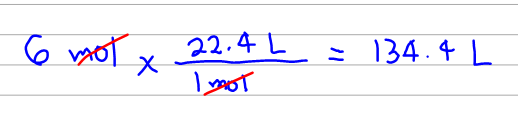# Problem: If 6.00 mol of an ideal gas at STP were confined to a cube, what would be the length in cm of an edge of this cube?

🤓 Based on our data, we think this question is relevant for Professor Sumner's class at UF.

###### FREE Expert Solution

The first thing we need to know here is that at STP, 1 mole of an ideal gas will have a volume of 22.4 L. So we can simply calculate the volume of 6 moles as:Now we need to convert the units from L to cm3  to have the proper units for the dimensions that we'll need later to compute for the edge of the cube.###### Problem Details

If 6.00 mol of an ideal gas at STP were confined to a cube, what would be the length in cm of an edge of this cube?